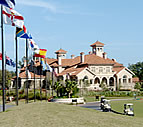×

# fraction

1 ENTRIES FOUND:
fraction /ˈfrækʃən/ noun
plural fractions
fraction
/ˈfrækʃən/
noun
plural fractions
Learner's definition of FRACTION
[count]
mathematics : a number (such as ¹/₂ or ³/₄) which indicates that one number is being divided by another
also : a number (such as 3.323) that consists of a whole number and a decimal
: a part or amount of something